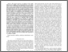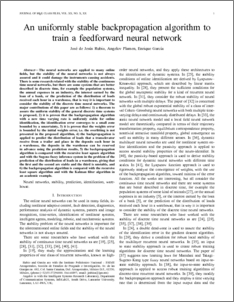# An uniformly stable backpropagation algorithm to train a feedforward neural network

Rubio, José de Jesús and Angelov, Plamen and García, Enrique (2011) An uniformly stable backpropagation algorithm to train a feedforward neural network. IEEE Transactions on Neural Networks, 22 (3). pp. 356-366. ISSN 1045-9227Preview
PDF (10IEEETNN_(2).pdf)
10IEEETNN_(2).pdf

## Abstract

The neural networks are applied to many online elds, but the stability of the neural networks is not always assured and it could damage the instruments causing accidents. There is some research related with the stability of the continuous time neural networks, but there are some systems that are better described in discrete time, for example the population systems, the annual expenses in an industry, the interest earned by the loan of a bank, or the prediction of the distribution of loads received each hour in a warehouse, that is way it is important to consider the stability of the discrete time neural networks. The major contributions of this paper are as follows: 1) a theorem to assure the uniform stability of the general discrete time systems is proposed, 2) it is proven that the backpropagation algorithm with a new time varying rate is uniformly stable for online identication, the identication error converges to a small zone bounded by a uncertainty, 3) it is proven that the weights error is bounded by the initial weights error, i.e. the overtting is not presented in the proposed algorithm, 4) the backpropagation is applied to predict the distribution of loads that a transelevator receive from a trailer and place in the deposits each hour in a warehouse, the deposits in the warehouse can be reserved in advance using the prediction results, 5) the backpropagation algorithm is compared with the recursive least square algorithm and with the Sugeno fuzzy inference system in the problem of the prediction of the distribution of loads in a warehouse, giving that the rst and the second are stable and the third is unstable, and 6) the backpropagation algorithm is compared with the recursive least square algorithm and with the Kalman lter algorithm in an academic example. (c) IEEE Press

Item Type:
Journal Article
Journal or Publication Title:
IEEE Transactions on Neural Networks
Uncontrolled Keywords:
/dk/atira/pure/researchoutput/libraryofcongress/qa75
Subjects:
ID Code:
34354
Deposited By:
Deposited On:
17 Jan 2011 16:08
Refereed?:
Yes
Published?:
Published